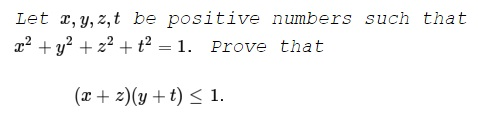# An Inequality from Gazeta Matematica, March 2016 II

### Problem

Several inequalities with solution by Dan Sitaru and Leo Giugiuc have been just published in Gazeta Matematica (March 2016). The simplest of these is discussed on a separate page. So far it gathered more than ten solutions. I'll try to partially reproduce some of that experience.### Proof 1

Define matrix $\displaystyle A=\left(\begin{array}\;x&y&z&t\\t&x&y&z\end{array}\right).\;$ We have

\displaystyle\begin{align} A\cdot A^t &= \left(\begin{array}\;x&y&z&t\\t&x&y&z\end{array}\right)\cdot \left(\begin{array}\;x&t\\y&x\\z&y\\t&z\end{array}\right)\\ &=\left(\begin{array}\;x^2+y^2+z^2+t^2 & xt+xy+zy+tz\\xt+xy+zy+tz & x^2+y^2+z^2+t^2\end{array}\right)\\ &=\left(\begin{array}{c,c}\;1 & (x+z)(y+t)\\(x+z)(y+t) & 1\end{array}\right). \end{align}

By Cauchy-Binet theorem, $\text{det}(A\cdot A^t)\ge 0.\;$ Therefore, $[(x+z)(y+y)]^2\le 1^2,\;$ or, $(x+z)(y+y)\le 1.$

### Proof 2

$(x-y)^2+(y-z)^2+(x-t)^2+(y-t)^2\ge 0\;$ which simplifies to $x^2+y^2+z^2t^2-xy-yz-xt-yt\ge 0.\;$ This is exactly $(x+z)(y+t) \le 1.$

### Proof 3

By the AM-QM inequality, $\displaystyle\frac{x+y+z+t}{4}\le\sqrt{\frac{x^2+y^2+z^2+t^2}{4}}=\frac{1}{2}\;$ so that $x+y+z+t\le 2.\;$ Further, by the AM-GM inequality,

\displaystyle\begin{align} (x+z)(y+t) &\le \left(\sqrt{\frac{x+z+y+t}{2}}\right)^2\\ &\le \left(\sqrt{\frac{2}{2}}\right)^2\\ &=1. \end{align}

### Proof 4

From $(x+z)^2\le 2(x^2+z^2)\;$ and $(y+t)^2\le 2(y^2+t^2)\;$ we obtain a sequence of inequalities:

\displaystyle\begin{align} (x+z)^2(y+t)^2 &\le 4(x^2+z^2)(y^2+t^2)\\ &\le 4\left(\frac{x^2+z^2+y^2+t^2}{2}\right)^2\\ &= 4\left(\frac{1}{2}\right)^2\\ &= 4\cdot \frac{1}{4}. \end{align}

and, therefore, $(x+z)(y+t)\le 1.$

### Proof 5

Use Lagrange multipliers to prove that

$\displaystyle\max_{x^2+y^2+z^2+t^2=1}(x+z)(y+t)=1.$

Let $J=(x+z)(y+t)+\lambda (x^2+y^2+z^2+t^2-1).$

Taking $\displaystyle\frac{\partial J}{\partial x}=\frac{\partial J}{\partial y}=\frac{\partial J}{\partial z}=\frac{\partial J}{\partial t}=\frac{\partial J}{\partial\lambda}=0\;$ yields

$y+t+2x\lambda=0,\\ x+z+2y\lambda=0,\\ y+t+2z\lambda=0,\\ x+z+2t\lambda=0,\\ x^2+y^2+z^2+t^2=1.$

It follows that $x=z\;$ and $y=t.\;$ So that $2(x^2+y^2)=1\;$ and we need to find $\max 2x\cdot 2y.\;$ But, since $2xy\le x^2+y^2,\;$ $4xy\le 2(x^2+z^2)=1,$ with the equality only when $x=y.$

To sum up, $\displaystyle\max_{x^2+y^2+z^2+t^2=1}(x+z)(y+t)=1,$ with the maximum achieved for $\displaystyle x=y=z=t=\frac{1}{2}.$

### Proof 6

Sk Rejuan used, as before, the Cauchy-Schwarz inequality:

\begin{align} [(x+z)(y+t)]^2 &= [xy+yz+zt+tx]^2\\ &\le(x^2+y^2+z^2+t^2)(y^2+z^2+t^2+x^2)\\ &=1. \end{align}

### Proof 7

Daniel Liu put the AM-QM inequality into the fast lane:

$\displaystyle (x+z)(y+t)\le\frac{(x+y+z+t)^2}{4}\le x^2+y^2+z^2+t^2=1.$

### Linear Algebra Tools for Proving Inequalities

$\;\left(\displaystyle\left(\frac{a}{b-c}\right)^2+\left(\frac{b}{c-a}\right)^2+\left(\frac{c}{a-b}\right)^2\ge 2\right)$
• Linear Algebra Tools for Proving Inequalities: Cauchy-Binet Formula $\;\left(\displaystyle\left(\sum_{i=1}^{n}\frac{x_i^2}{a_i}\right)\cdot\left(\sum_{1\le i\lt j\le n}a_ia_j(x_iy_j-x_jy_i)^2\right)\ge \sum_{i=1}^{n}a_iy_i^2\right)$
• An Inequality from Gazeta Matematica, March 2016 (If $a^2+b^2+c^2=3\,$ then $(a+c)(1+b)\le 4)$
• An Inequality from Gazeta Matematica, March 2016 III $\;(a^2+b^2+1\ge a+ab+b)$
• An Inequality from Gazeta Matematica, March 2016 IV (If $a^2+b^2+c^2=1\,$ then $a+ac+b\le 2)$
• Problem 3980 from Crux Mathematicorum $\;\left(\displaystyle\sum_{cycl}\frac{a+b}{a-b}\prod_{cycl}\frac{a+b}{a-b}\lt\frac{1}{3}\right)$
• NonSquare Matrix as a Tool for Proving an Inequality $\;\left(2(a + b + c)((a + 2b + 3c) \ge (\sqrt{b(a+b)} + 2\sqrt{c(b+c)} + \sqrt{a(c+a)})^2\right)$
• An Inequality in Parallelogram of Unit Area $\;\left(a^2+b^2+c^2+d^2+ac+bd\ge\sqrt{3}\right)$
• An Inequality from a Vietnamese Problem Book $\;\left(\displaystyle \frac{a^3+2}{b+2c}+\frac{b^3+2}{c+2a}+\frac{c^3+2}{a+2b}\ge 3\right)$
• Hadamard's Determinant Inequalities and Applications I $\left((2-a-b-c+abc)^2\le (a^2+2)(b^2+2)(c^2+2)\right)$
• Hadamard's Determinant Inequalities and Applications II $\left((n + a - 1)(a - 1)^{n-1} \le a^n\right)$
•